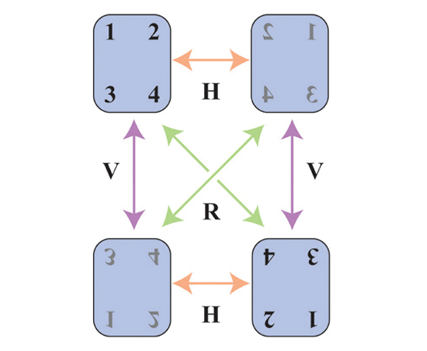Birds migrate south in the fall. Squirrels gather nuts. Humans also have behavioral rituals in the autumn. I change the batteries in my smoke detectors, I switch my clocks back to daylight standard time, and I turn the mattress on my bed. The first two are relatively easy. There's even a mnemonic for changing the clocks to and from daylight savings time: "spring forward, fall back."

Man-handling a heavy, queen-size mattress can be a challenge, but it is important so that your mattress wears evenly. There are four positions that a mattress can be in, and it is important to use all four positions over a two-year cycle. I use a simple mnemonic to ensure that I alternate between all four positions: "spring spin, fall flip."

How did I develop this mnemonic? By using matrices, of course!

### Mattress positions

There are three ways to turn a mattress:

• The rotation (R): This is the easiest maneuver, and it requires only one person. It doesn't flip the mattress, but spins it on the box spring so that the head becomes the foot. (When an airplane rotates along this axis, the movement is called "yaw.")
• The horizontal flip (H): This is the easiest way to flip a mattress from one side to the other. It requires that you turn the mattress along its long axis. (When an airplane rotates along this long axis, the movement is called "roll.")
• The vertical flip (V): This is the hardest way to flip a mattress, and might be impossible if you have low ceilings! It requires that you turn the mattress along its short axis. (When an airplane rotates along this short axis, the movement is called "pitch.")

Fortunately, it turns out that you NEVER need to use the vertical flip! You can get even wear on your mattress by alternating the two easier moves. In the spring, use the rotation; in the fall, use the horizontal flip. "Spring spin, fall flip." Repeat this every year and your mattress will mathe-magically alternate between all four possible positions.

### The matrices of mattresses

Rotations can be represented by the actions of matrices. Set up a coordinate axis in the middle of your mattress so that the y axis points towards the head of the bed and the z axis points toward the ceiling. Then the rotation (R) sends a vector (x, y, z) to the new vector (-x, -y, z). Similarly, the horizontal flip (H) send (x, y, z) to (-x, y, -z), and the vertical flip (V) send (x, y, z) to (x, -y, -z). This means that the three rotations can be represented by the following matrices:

```proc iml; /* R: rotation about the z axis = rotate 180 degrees in the (x,y)-plane */ R = {-1 0 0, 0 -1 0, 0 0 1};   /* H: rotation about the y axis = rotate 180 degrees in the (x,z)-plane */ H = {-1 0 0, 0 1 0, 0 0 -1};   /* V: rotation about the x axis = rotate 180 degrees in the (y,z)-plane */ V = { 1 0 0, 0 -1 0, 0 0 -1};```

These matrices have a few interesting properties. The first is that they are their own inverses: H*H = V*V = R*R = I. The second is that you can use any two to obtain the third. In particular, R*H=V. This is great, because it means that you don't ever need to attempt the difficult vertical flip, you can use the product of simpler rotations to achieve the same result.

### A previous mattress article

When I started writing this article, I searched the Internet for an illustration that I could use. I discovered a wonderful article that contains some excellent illustrations and much of the same content. The author is my fellow Cornellian, Steve Strogatz, and he wrote it for the New York Times . (By the way, if you haven't read Strogatz's book, Synch, I highly recommend it. He also gave an entertaining 20-minute TED talk on the subject of "synch.")

The Strogatz article illustrates the mattress rotations with the following colorful diagram, which shows the four possible positions of a mattress and how each rotation takes you from one position to another:Suppose that your mattress is initially in the position in the upper left corner. Let's see what happens if you follow "spring spin, fall flip" for two consecutive years. That is, you rotate the mattress according to the sequence R, followed by H, followed by R, followed by H:

1. After the first "spring spin," the mattress position is as shown in the lower right of the diagram.
2. A "fall flip" moves the mattress into the position showed in the lower left.
3. After a second "spring spin," the mattress position is as shown in the upper right.
4. A second "fall flip" returns the mattress to its original position.

These matrix representations are not the only ones possible. You can also represent the rotations by using permutation matrices, which I've discussed in a previous article on gift-giving among family members. A permutation matrix acts on the vector (1,2,3,4) and maps the four positions of the mattress to each other. One permutation matrix is H = {0 1 0 0, 1 0 0 0, 0 0 0 1, 0 0 1 0}; I'll leave the construction of the R and V permutation matrices as an exercise.

ShareDistinguished Researcher in Computational Statistics

Rick Wicklin, PhD, is a distinguished researcher in computational statistics at SAS and is a principal developer of PROC IML and SAS/IML Studio. His areas of expertise include computational statistics, simulation, statistical graphics, and modern methods in statistical data analysis. Rick is author of the books Statistical Programming with SAS/IML Software and Simulating Data with SAS.

2.3.